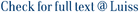We study the following backward stochastic differential equation on finite time horizon driven by an integer-valued random measure ц on R+ × E, where E is a Lusin space, with compensator v(dt, dx) = dAt Øt(dx): The generator f satisfies, as usual, a uniform Lipschitz condition with respect to its last two arguments. In the literature, the existence and uniqueness for the above equation in the present general setting has only been established when A is continuous or deterministic. The general case, i.e. A is a right-continuous nondecreasing predictable process, is addressed in this paper.

Existence and uniqueness for BSDEs driven by a general random measure, possibly non quasi-left-continuous / Bandini, Elena. - In: ELECTRONIC COMMUNICATIONS IN PROBABILITY. - ISSN 1083-589X. - 20:71(2015), pp. 1-13. [10.1214/ECP.v20-4348]

### Existence and uniqueness for BSDEs driven by a general random measure, possibly non quasi-left-continuous

#### Abstract

We study the following backward stochastic differential equation on finite time horizon driven by an integer-valued random measure ц on R+ × E, where E is a Lusin space, with compensator v(dt, dx) = dAt Øt(dx): The generator f satisfies, as usual, a uniform Lipschitz condition with respect to its last two arguments. In the literature, the existence and uniqueness for the above equation in the present general setting has only been established when A is continuous or deterministic. The general case, i.e. A is a right-continuous nondecreasing predictable process, is addressed in this paper.
##### Scheda breve Scheda completa Scheda completa (DC)2015
Backward stochastic differential equations; Random measures; Statistics and Probability; Statistics, Probability and Uncertainty
Existence and uniqueness for BSDEs driven by a general random measure, possibly non quasi-left-continuous / Bandini, Elena. - In: ELECTRONIC COMMUNICATIONS IN PROBABILITY. - ISSN 1083-589X. - 20:71(2015), pp. 1-13. [10.1214/ECP.v20-4348]
File in questo prodotto:
File
BSDE_Published.pdf

Open Access

Tipologia: Versione dell'editore
Licenza: DRM (Digital rights management) non definiti
Dimensione 268.7 kB
Utilizza questo identificativo per citare o creare un link a questo documento: `https://hdl.handle.net/11385/172158`
•16
•15